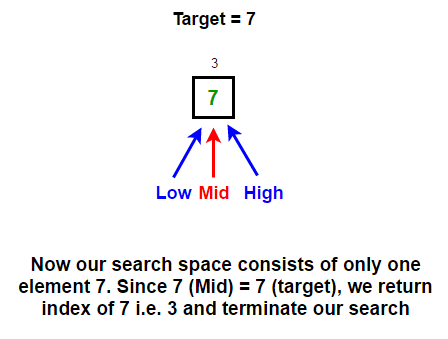# Binary Search Algorithm | Recursive & Iterative Implementation

Given a sorted array of integers and a target value, find out if a target exists in the array or not in O(log(n)) time using Binary Search Algorithm in C and Java. If target exists in the array, print index of it.

For example,

Input:
arr[] = [2, 3, 5, 7, 9]
target = 7

Output: Element found at index 3

Input:
arr[] = [1, 4, 5, 8, 9]
target = 2

A simple solution would be to perform Linear search on the given array. It sequentially checks each element of the array for the target value until a match is found or until all the elements have been searched. Worst case time complexity of this approach is O(n) as it makes at most n comparisons, where n is the length of the array. This approach don’t take advantage of the fact that array is sorted.

#### How can we perform better?

The idea is to use Binary Search. Binary Search is a divide and conquer algorithm. Like all divide and conquer algorithms, Binary Search first divides a large array into two smaller sub-arrays and then recursively (or iteratively) operate the sub-arrays. But instead of operating on both sub-arrays, it discards one sub-array and continue on the second sub-array. This decision of discarding one sub-array is made in just one comparison.

So Binary Search basically reduces the search space to half at each step. By search space we mean sub-array of given array where the target value is located (if present in the array). Initially, the search space is the entire array and binary search redefine the search space at every step of the algorithm by using the property of the array that it is sorted. It does so by comparing the mid value in the search space to the target value. If the target value matches the middle element, its position in the array is returned else it discards half of the search space based on the comparison result.

Let us track the search space by using two index – start and end. Initially, start = 0 and end = n-1 (as initially whole array is our search space). At each step, we find the mid value in the search space and compares it with target value. There are three cases possible –

Case 1: If target = A[mid], we return mid.

Case 2: If target < A[mid], we discard all elements in the right search space including the mid element i.e. A[mid..high]. Now our new high would be mid – 1.

Case 3: target > A[mid], we discard all elements in the left search space including the mid element i.e. A[low..mid]. Now our new low would be mid + 1.

We repeat the process until target is found or our search space is exhausted. Let’s understand this by taking a example. Let

arr = [2, 3, 5, 7, 8, 10, 12, 15, 18, 20]
target = 7## Java

Output:

Element found at index 1

We can easily convert above iterative version of the binary search algorithm to recursive one. Below is the recursive version.

## Java

Output:

Element found at index 1

### Performance of Binary Search Algorithm:

We know that at each step of the algorithm, our search space reduces to half. That means if initially our search space contains n elements, then after one iteration it contains n/2, then n/4 and so on..

n -> n/2 -> n/4 -> … -> 1

Suppose after k steps our search space is exhausted. Then,

n/2k = 1
n = 2k
k = log2n

Therefore, time complexity of binary search algorithm is O(log2n) which is very efficient. Auxiliary space used by it is O(1) for iterative implementation and O(log2n) for recursive implementation due to call stack.

### Avoid Integer Overflow:

signed int in C/C++ takes up 4 bytes of storage i.e. It allows storage for integers between -2147483648 to 2147483647 (Note that some compilers might take up 2 bytes storage as well. The exact value can be find by cout << sizeof(int)).

So if (low + high) > 2147483647, integer overflow will happen.

int mid = (low + high)/2; // overflow can happen

To avoid integer overflow, we can use any of the below expressions –

int mid = low + (high – low)/2; OR
int mid = high – (high – low)/2;

Now, low + (high – low) / 2 or high – (high – low) / 2 always computes a valid index halfway between high and low, and overflow will never happen even for extreme values.(3 votes, average: 5.00 out of 5)Loading...

Please use our online compiler to post code in comments. To contribute, get in touch with us.
Like us? Please spread the word and help us grow. Happy coding 🙂Subscribe
Notify ofGuest

> Auxiliary space used by it is O(1).

This is technically false, you use recursion so you need space for the stack frames, so the auxiliary space you are using is equal to max recursion depth. If splitting in 2, this means same as time complexity – O(log_2 n)Guest
John Smith

the second picture is false? The mid should be A?